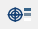# Calculate Linearized Stress

Decompose a through-thickness elastic stress field into equivalent membrane, bending, and peak stresses for comparison with appropriate allowable limits.

1. On the Project Tree, open the Analysis Workbench.
2. Click(Pick info).
3. In the dialog, select the Linearized stress tab.
4. Optional: Enter the failure criteria for Membrane stress, Membrane + bending stress and Peak stress to see the pass/fail status of the created segment. The default values are calculated using the material’s yield stress.

Membrane stress = 2/3 of Yield stress

Membrane + bending stress = Yield stress

Peak stress = 2x Yield stress

When multiple materials are present in the model, the material with highest yield stress is used to calculate default allowables.

5. Under Stress linearization segment, define the line segment in one of the following ways:
Option Steps
Normal to surface Activate the Normal to surface radio button.

SimSolid launches a ray which is normal to the surface and passes through the part.

Custom
1. Activate the Custom radio button.
2. In the modeling window, pick the entry and exit points on the part.

The entry and exit point coordinates are populated in the dialog.

Normal to datum points
1. Activate the Normal to datum points radio button.
2. In the drop-down menu, select a point set.
Linearized local stresses are evaluated on the fly and posted in the dialog for each line segment defined. By default, invariants are displayed in the table.
6. Select the Show linearized stress tensor components check box to view stress tensor components along with invariants.
Tip: Status of the line shows passed or failed based on the failure criteria. Failure criteria is only evaluated on the invariants.
7. Optional: Save linearized stress information as a CSV file.
1. Click Save to CSV.
2. In the dialog, enter a file name and select a save location.
3. Click Save.

## Calculation Method

SimSolid method for calculating linearized stress.

Linearized stress decomposes a through-thickness elastic stress field into equivalent membrane, bending, and peak stresses for comparison with appropriate allowable limits.

Linearized stress is calculated as follows:
1. The stress is extracted by interpolation in a local coordinate system at all the points along the line. The local coordinate system is based on the start and end points of the stress linearization segment.
The X-axis of the system is along the segment from entry to exit points. The other two axes are calculated as follows:
• If the local x-axis is not parallel to the global y-axis:

Zlocal = Xlocal x Yglobal

Ylocal = Zlocal x Xlocal

• If the local x-axis is parallel to the global y-axis:

local y-axis (Ylocal) is negative of global-x if local-x is along positive global-y, and vice versa.

Zlocal = Xlocal x Ylocal

2. From the extracted stress values above, the average membrane stress tensor + bending stress tensors at the entry and exit points are calculated using numerical integration.

${\sigma }_{i}^{m}\text{\hspace{0.17em}}=\text{\hspace{0.17em}}\frac{1}{L}{\int }_{-L/2}^{L/2}{\sigma }_{i}dx$

${\sigma }_{iS}^{b}\text{\hspace{0.17em}}=\text{\hspace{0.17em}}-\frac{6}{{L}^{2}}{\int }_{-\frac{L}{2}}^{\frac{L}{2}}{\sigma }_{i}xdx$

${\sigma }_{iE}^{b}\text{\hspace{0.17em}}=\text{\hspace{0.17em}}-{\sigma }_{iS}^{b}$

${\sigma }_{i}^{m}$ = ith component of membrane stress

${\sigma }_{i}$ = ith component of extracted stress value

${\sigma }_{iS}^{b}$ = ithcomponent of bending stress at the entry

${\sigma }_{iE}^{b}$ = ith component of bending stress at the exit

L = Length of the Stress linearization segment

x = position of a point along the segment

3. Peak stress and membrane and bending stress are also calculated at the entry and exit.

${\sigma }_{iS}^{p}\text{\hspace{0.17em}}=\text{\hspace{0.17em}}{\sigma }_{iS}\text{\hspace{0.17em}}-\text{\hspace{0.17em}}\left({\sigma }_{i}^{m}\text{\hspace{0.17em}}+\text{\hspace{0.17em}}{\sigma }_{iS}^{b}\right)$

${\sigma }_{iE}^{p}\text{\hspace{0.17em}}=\text{\hspace{0.17em}}{\sigma }_{iE}\text{\hspace{0.17em}}-\text{\hspace{0.17em}}\left({\sigma }_{i}^{m}\text{\hspace{0.17em}}+\text{\hspace{0.17em}}{\sigma }_{iE}^{b}\right)$

${\sigma }_{iS}^{p}$ = ith component of peak stress at the entry

${\sigma }_{iE}^{p}$ = ith component of peak stress at the exit

4. Finally, invariants for the membrane, membrane + bending, and peak stresses are calculated.